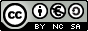Multiples and factors. Prime numbers First and Second Year of Secondary Education

Composite or prime number.

A prime number is a natural number that has exactly two factors: itself and 1.
A composite number is a natural number that has more than two factors. It is obtained as the product of prime numbers.

Esta unidad interactiva requiere la máquina virtual de Java J2RE.
 Identify if a number is prime or composite. Type any number in the control box below and hit Enter, then you can  change the value of the number by using the up and down triangles. You will be shown if this number is prime or composite. If it is composite, you will also be shown which number you can divide it by, apart from 1. The largest number you can type is 10.000.000.000

Investigate:

- From number 2, using the up triangle, go up one at the time and count the prime numbers in the first hundred: from 2 to 101.
- Now type a number of 9 digits, start with one ending in 00, go up one at the time and count the prime numbers in this hundred.
- What can you deduce from this?

 Eduardo Barbero CorralSpanish Ministry of Education. Year 2007